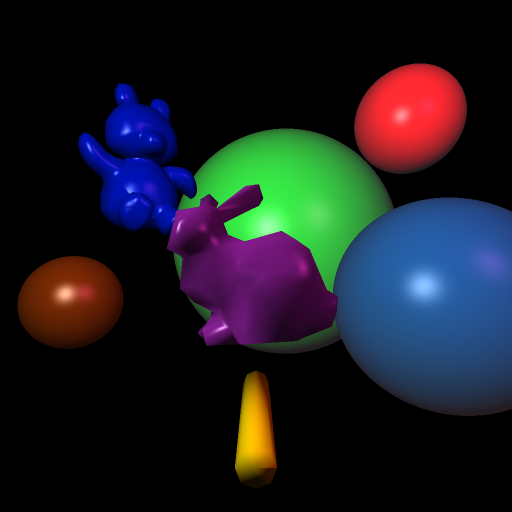# CS 636 Programming Assignment 3 - Add an acceleration technique

## Summary

Adding BVH acceleration reduced the rendering time from 192 to 4 seconds for the scene used for these images.

## Update on scene setup

After the first version of this I updated some of the scene setup from what is detailed below to make the objects brighter in the images, via the following changes:
```            Changed attenuation factor from 0.05 to 0.01

Changed ambient coefficient of bunny from 0.01 to 0.1
Changed diffuse coefficient of bunny from 0.3 to 0.5
Changed color of bunny from (0.7, 0.1, 0.6) to (0.8, 0.2, 0.9)
Changed rotation of bunny mesh from pi/4 to 0 so light shines directly on more of its surface.

Changed color of teddy from (0, 30/255, 108/255) to (0, 30/255, 255/255)
Changed ambient coefficient of teddy from 0.01 to 0.1
Changed diffuse coefficient of teddy from 0.3 to 0.8

Running times were 191 baseline and 4 for BVH acceleration so nearly identical to first version of scene.
```

## Scene Setup

This is similar to the scene from Assignment 2, but with two additional spheres added.

The camera for the scene is setup at (0,0,4) for the eye point, with a view direction of (0,0,-1) and a view up direction of (0, 1,0 ). The view plane distance is 6, and the horizontal camera angle is PI / 6.

The scene has two point light sources: a white light at (-5, 5, 5), and light source with color (0.4, 0.1, 0.3) rgb at position (13, 10, 5);

The scene contains 7 objects: four spheres and three mesh objects including a and a smooth shaded cylinder, a smooth shaded bunny, and a smooth shaded teddy bear.

### Bunny

The bunny is translated along the z axis +2 to move it closer to the camera from the original mesh in "bound-bunny_200.smf". It is also rotated 45 degrees around the Y axis.
• Diffuse surface color of purple (r,g,b) = (0.7, 0.1, 0.6)
• Specular surface color of white (r,g,b) = (1,1,1)
• Ambient surface color of purple (r,g,b) = (0.7, 0.1, 0.6)
• Diffuse coefficient of 0.3
• Specular coefficient of 0.7
• Ambient coefficient of 0.01
• Specular exponent of 30

### First Sphere

The first sphere (on the right) is centered at (8, -2, -10) with a radius of 4.5.
• Diffuse surface color of medium blue (r,g,b) = (3/255, 83/255, 151/255)
• Specular surface color of white (r,g,b) = (1,1,1)
• Ambient surface color of white (r,g,b) = (1,1,1)
• Diffuse coefficient of 0.8.
• Specular coefficient of 0.4
• Ambient coefficient of 0.1
• Specular exponent of 25

### Second Sphere

The second sphere (on the left) is centered at (-8, -2, -10) with a radius of 2.
• Surface color(diffuse and ambient) of orange (r,g,b) = (232/255, 99/255, 10/255)
• Specular surface color of white (r,g,b) = (1,1,1)
• Diffuse coefficient of 0.3.
• Specular coefficient of 0.6
• Ambient coefficient of 0.1
• Specular exponent of 15

### Third Sphere

The third sphere, the large one in the background, is centered at (2, 1, -20) with a radius of 8.
• Surface color(diffuse and ambient) of green (r,g,b) = (20/255, 252/255, 40/255)
• Specular surface color of white (r,g,b) = (1,1,1)
• Diffuse coefficient of 0.8.
• Specular coefficient of 0.4
• Ambient coefficient of 0.1
• Specular exponent of 25

### Fourth Sphere

The fourth sphere, the one to the top right, is centered at (9, 8, -15) with a radius of 3.
• Surface color(diffuse and ambient) of red (r,g,b) = (252/255, 20/255, 20/255)
• Specular surface color of white (r,g,b) = (1,1,1)
• Diffuse coefficient of 0.8.
• Specular coefficient of 0.4
• Ambient coefficient of 0.1
• Specular exponent of 25

The mesh for the teddy bear is translated by (-5, 4, -10), scaled by a factor of 0.15, and rotated around Y axis by PI / 4.

### Teddy Bear

• Surface color (diffuse and ambient) dark blue (r,g,b) = (0, 30/255, 108/255)
• Specular surface color of white (r,g,b) = (1,1,1)
• Diffuse coefficient of 0.5
• Specular coefficient of 0.5
• Ambient coefficient of 0.1
• Specular exponent of 25

### Cylinder

The cylinder is translated along the y axis -2 to move it lower down in the scene.
• Diffuse, specular, and ambient surface color of yellow (r,g,b) = (252/255, 217/255, 0)
• Diffuse coefficient of 0.8
• Specular coefficient of 0.05
• Ambient coefficient of 0.05
• Specular exponent of 2

## Original (non-accelerated) 512 by 512 Image

Original rendering time on my M1 Mac Mini was 190 seconds.## Rendering with BVH for acceleration 512 by 512 Image

Rendering time using BVH on my M1 Mac Mini was 4 seconds.